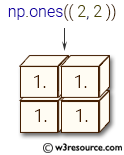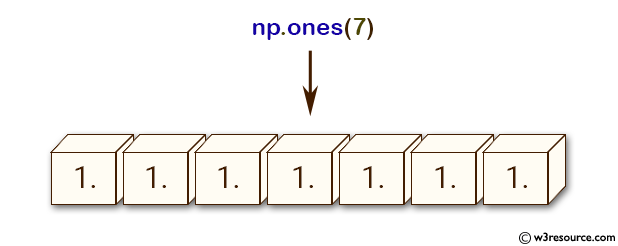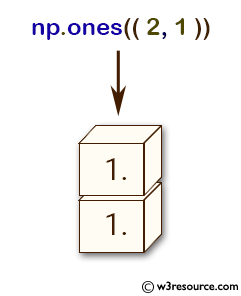# NumPy: numpy.ones() function

## numpy.ones() function

The numpy.ones() function is used to create a new array of given shape and type, filled with ones. The ones() function is useful in situations where we need to create an array of ones with a specific shape and data type, for example in matrix operations or in initializing an array with default values.

Syntax:

`numpy.ones(shape, dtype=None, order='C')`Parameters:

Name Description Required /
Optional
shape Shape of the new array, e.g., (2, 3) or 2. Required
dtype The desired data-type for the array, e.g., numpy.int8. Default is numpy.float64. optional
order Whether to store multi-dimensional data in row-major (C-style) or column-major (Fortran-style) order in memory optional

Return value:

[ndarray] Array of ones with the given shape, dtype, and order.

Example-1: Create arrays of ones with NumPy's ones() function

``````>>> import numpy as np
>>> np.ones(7)
array([ 1.,  1.,  1.,  1.,  1.,  1.,  1.])
>>> np.ones((2, 1))
array([[ 1.],
[ 1.]])
>>> np.ones(7,)
array([ 1.,  1.,  1.,  1.,  1.,  1.,  1.])
>>> x = (2, 3)
>>> np.ones(x)
array([[ 1.,  1.,  1.],
[ 1.,  1.,  1.]])
>>>``````

In the above code:

np.ones(7): This creates a 1-dimensional array of length 7 with all elements set to 1.

np.ones((2, 1)): This creates a 2-dimensional array with 2 rows and 1 column, with all elements set to 1.

np.ones(7,): This is equivalent to np.ones(7) and creates a 1-dimensional array of length 7 with all elements set to 1.

x = (2, 3) and np.ones(x): This creates a 2-dimensional array with 2 rows and 3 columns, with all elements set to 1.

Pictorial Presentation:Example: Createng a 3D array with ones along a specified axis

Code:

``````import numpy as np

# Create a 3D array with ones along the second axis
arr = np.ones((2, 3, 4), dtype=int)
arr[:, 1, :] = 2
print(arr)
``````

Output:

```[[[1 1 1 1]
[2 2 2 2]
[1 1 1 1]]

[[1 1 1 1]
[2 2 2 2]
[1 1 1 1]]]
```

In the above example, we create a 3D array with dimensions (2, 3, 4) using np.ones() function. We then set the values of the second axis to 2, resulting in a 3D array with ones along the first and third axis and 2's along the second axis.

Example: Create a 2D array with a custom data type and byte order

Code:

``````import numpy as np

# Create a 2D array of unsigned 16-bit integers with little-endian byte order
nums = np.ones((2, 3), dtype=np.dtype('<u2'))
print(nums)
``````

Output:

```[[1 1 1]
[1 1 1]]
```

In the above example, we create a 2D array with dimensions (2, 3) using np.ones() function. We specify the data type using np.dtype() function, which represents unsigned 16-bit integers with little-endian byte order. The resulting array has values of 1 and the specified data type and byte order.

Python - NumPy Code Editor:

Previous: identity()
Next: ones_like()

﻿